Case Based Questions (MCQ)

Chapter 13 Class 12 Probability
Serial order wise

## Based on the above data, answer any four of the  following questions.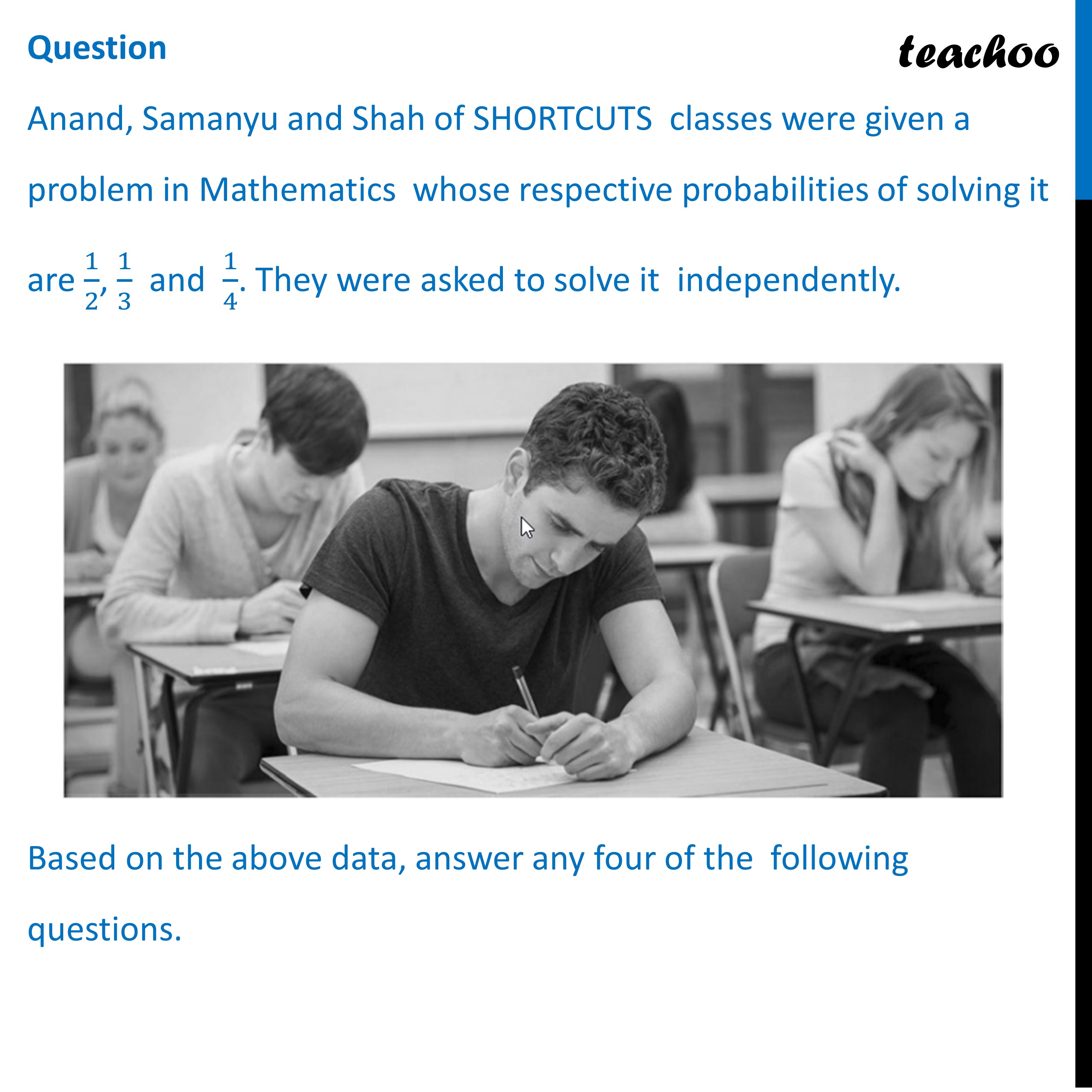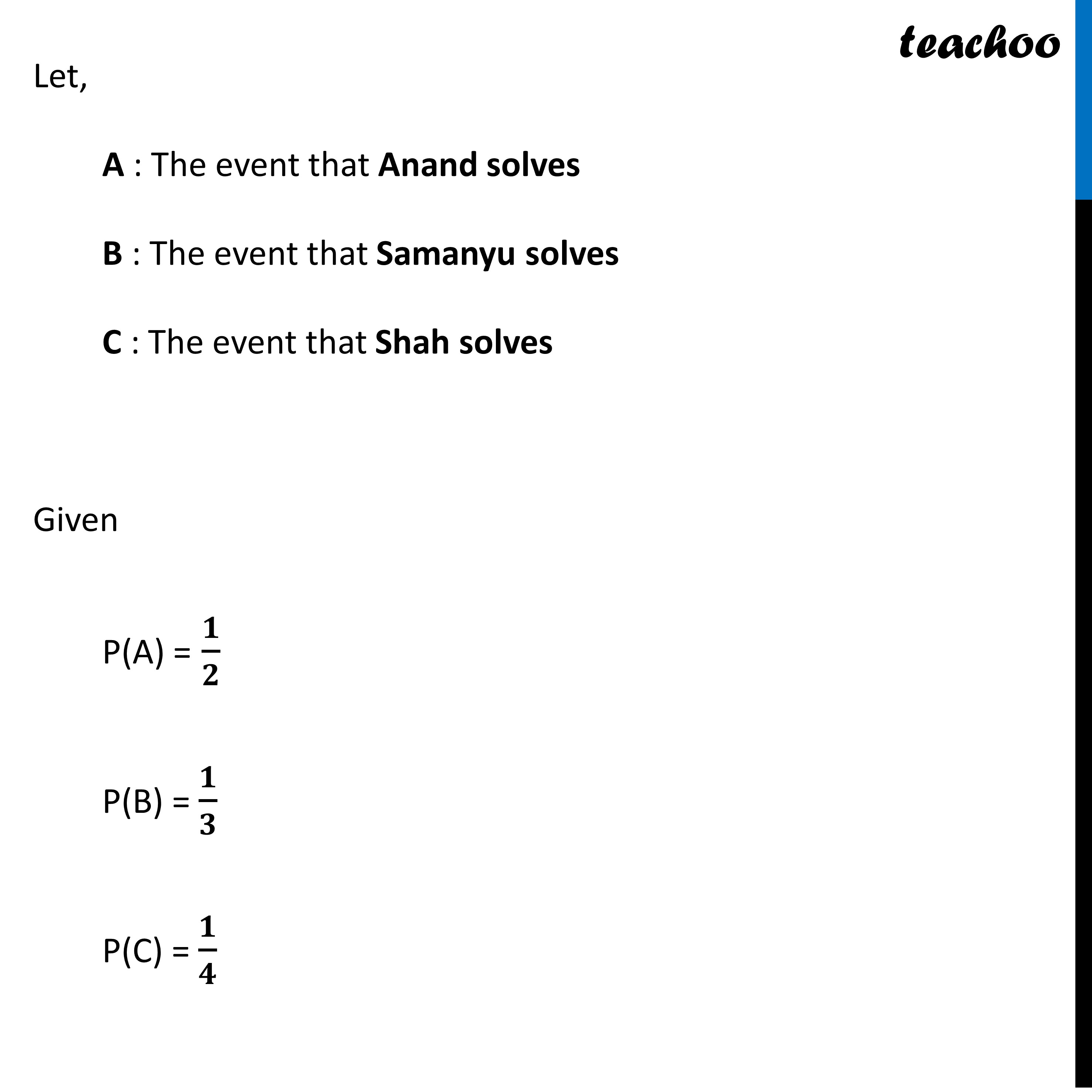## (D) 17/24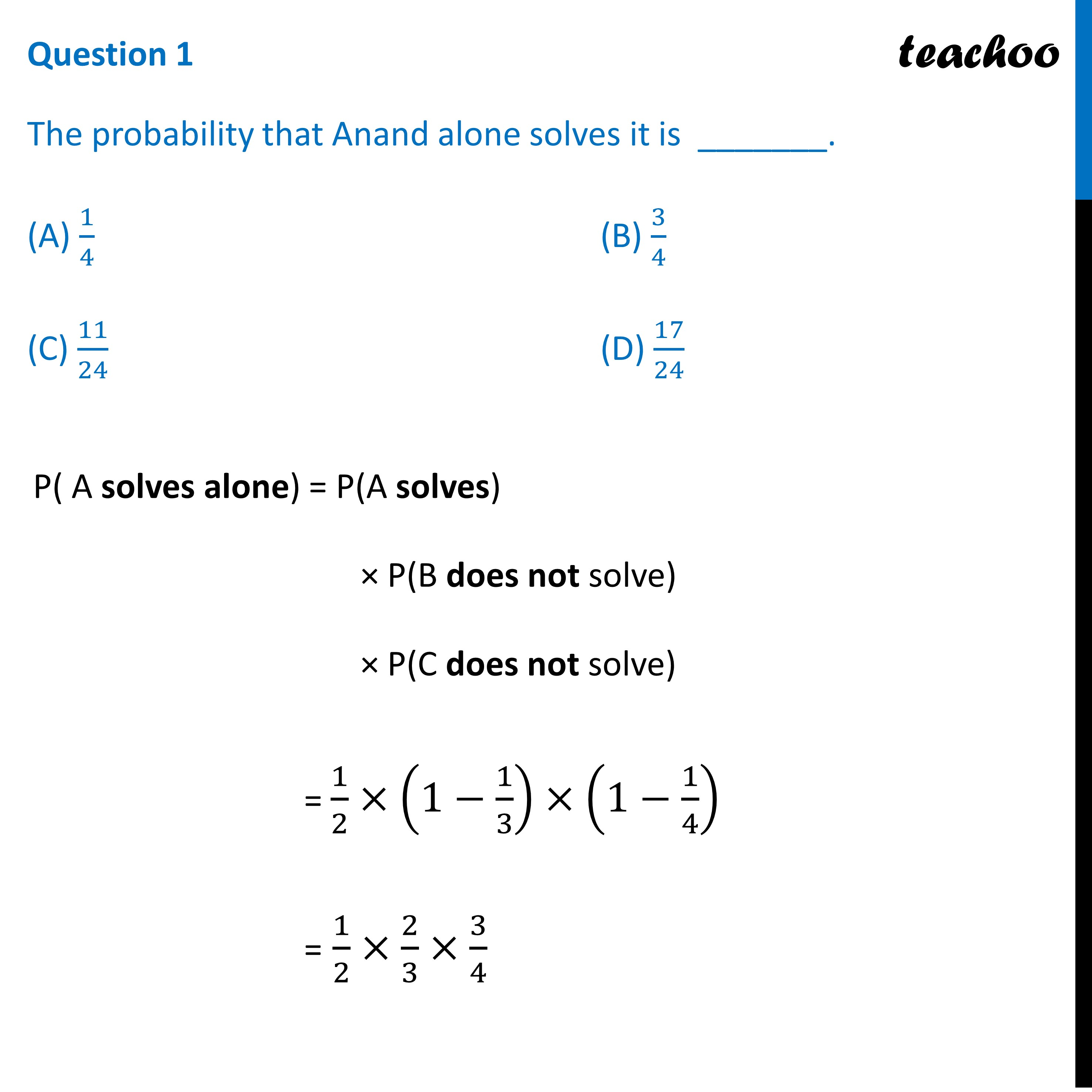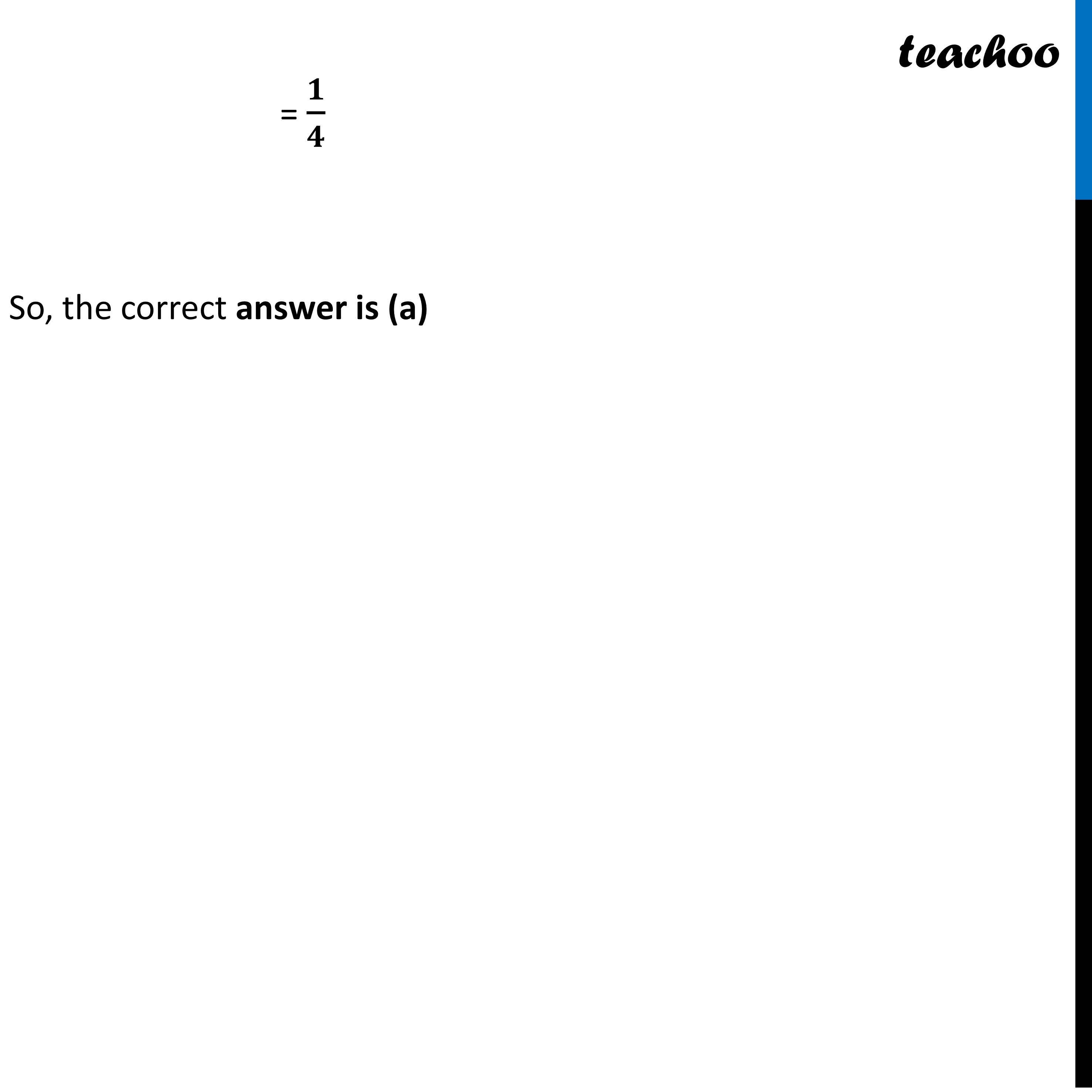## (D) 11/24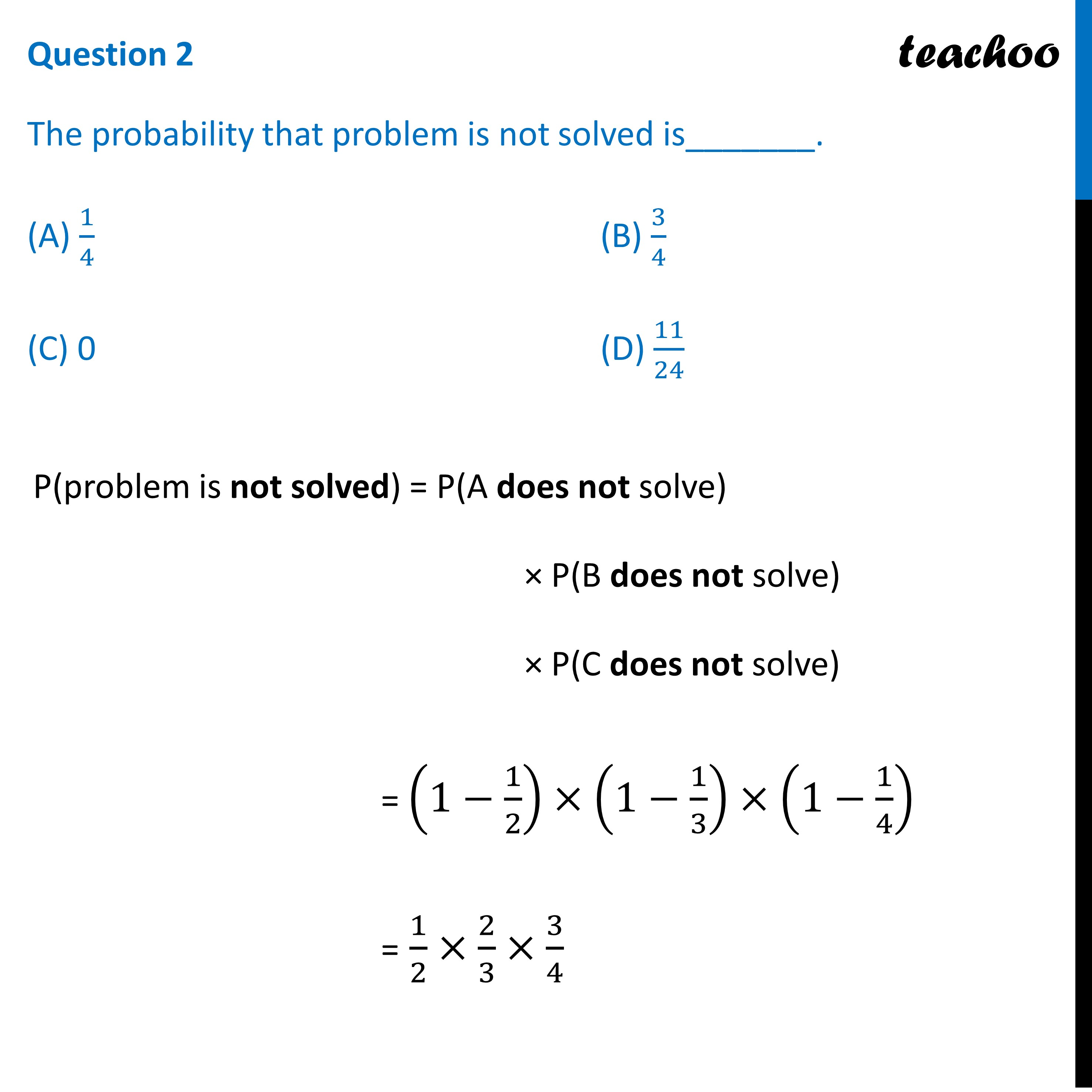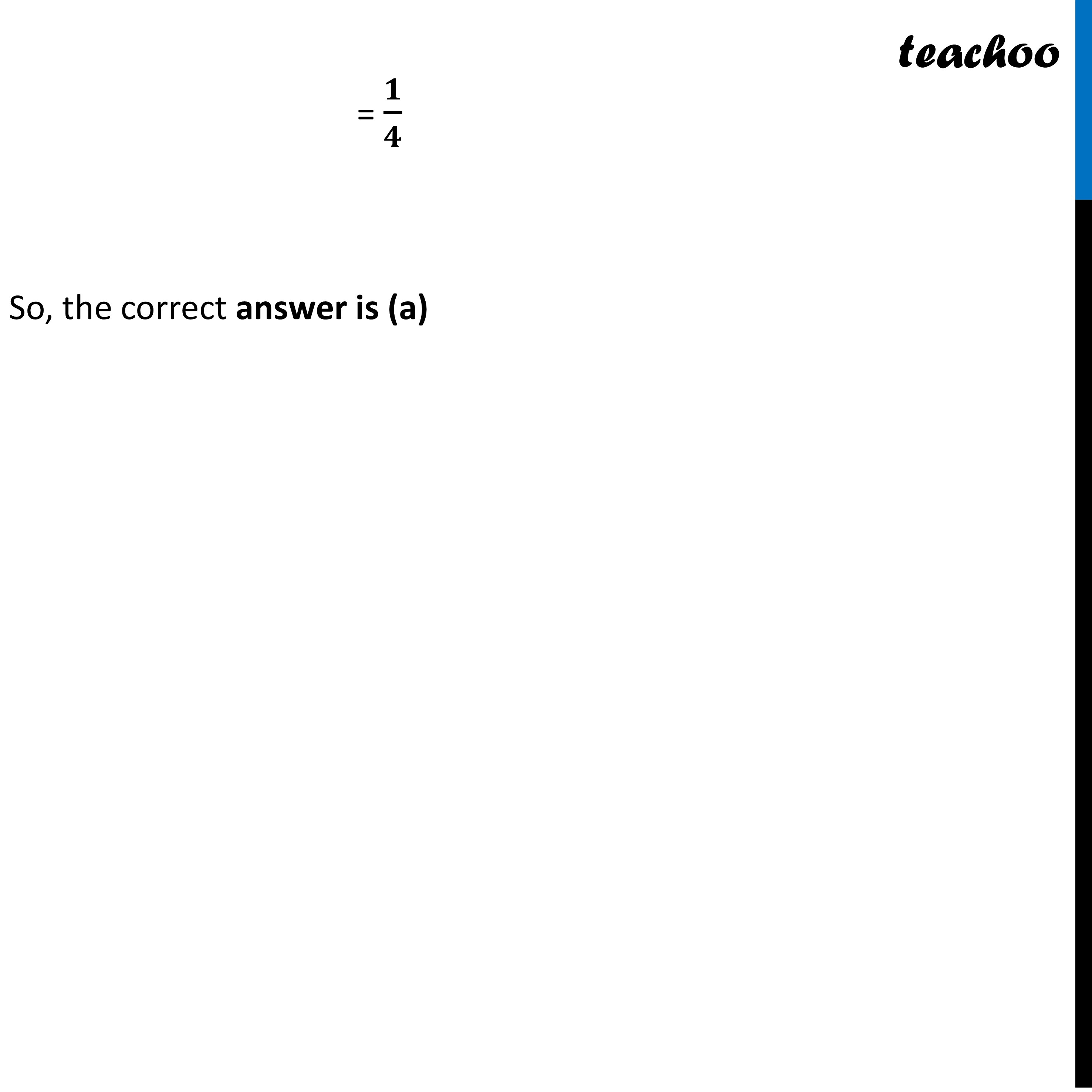## (D) 11/24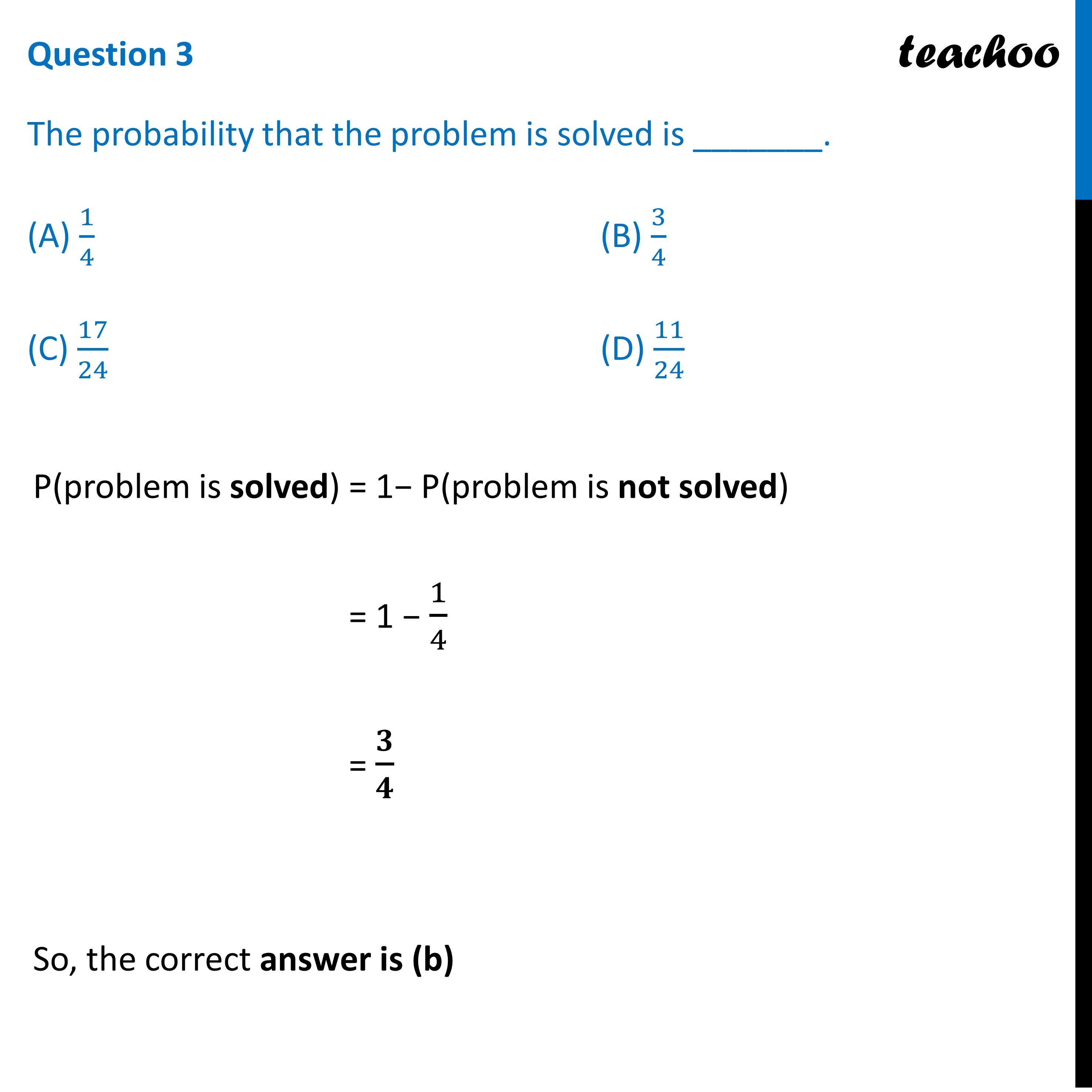## (D) 11/24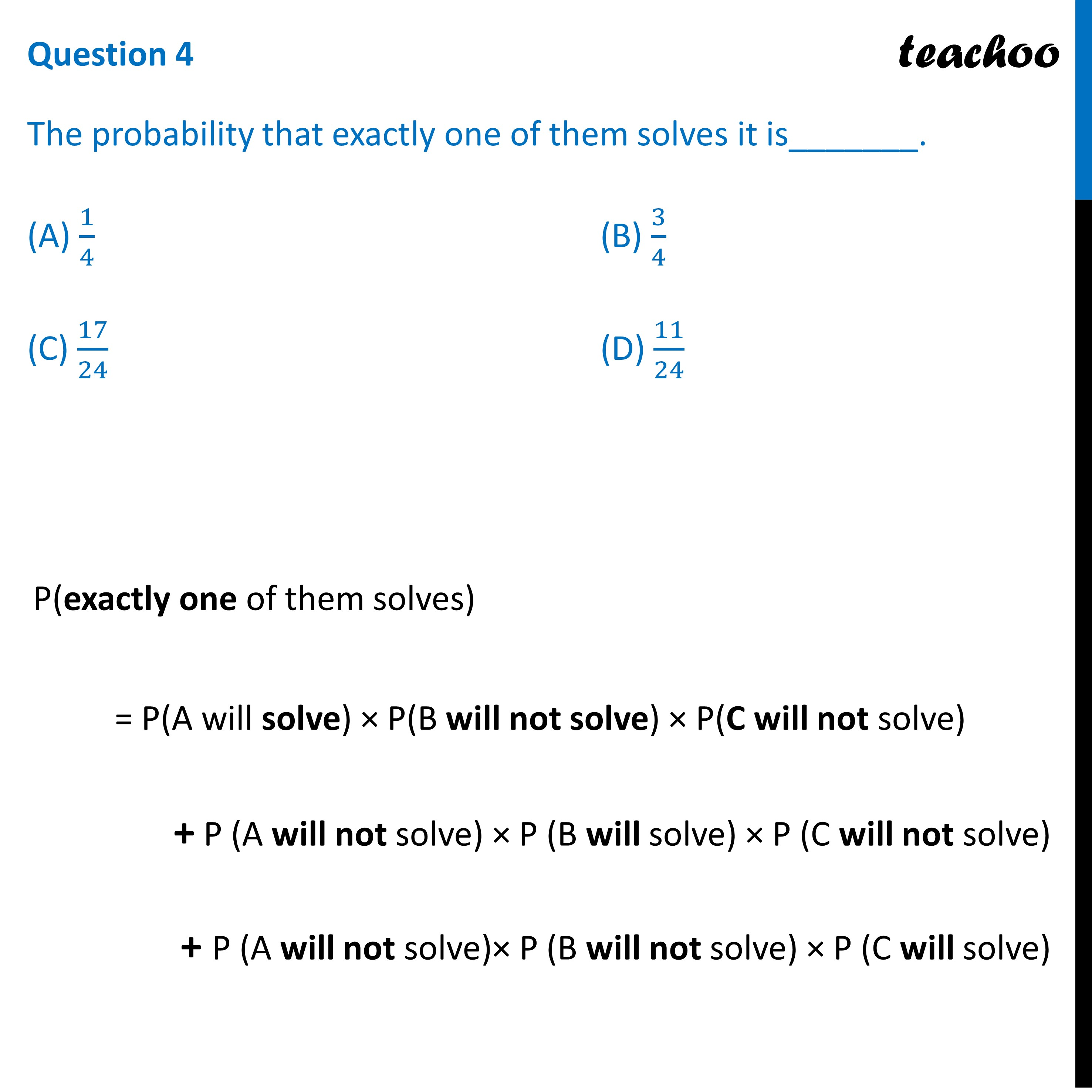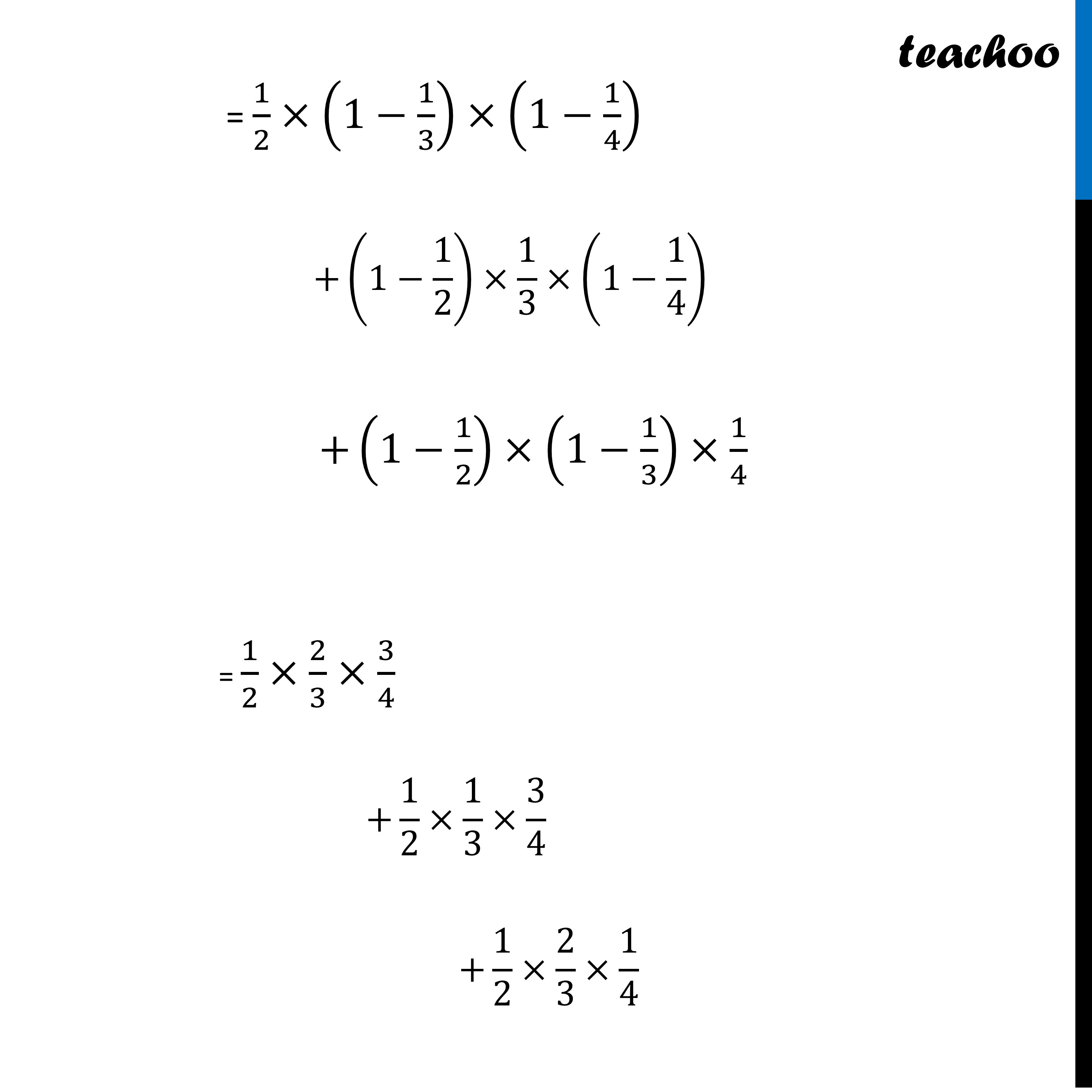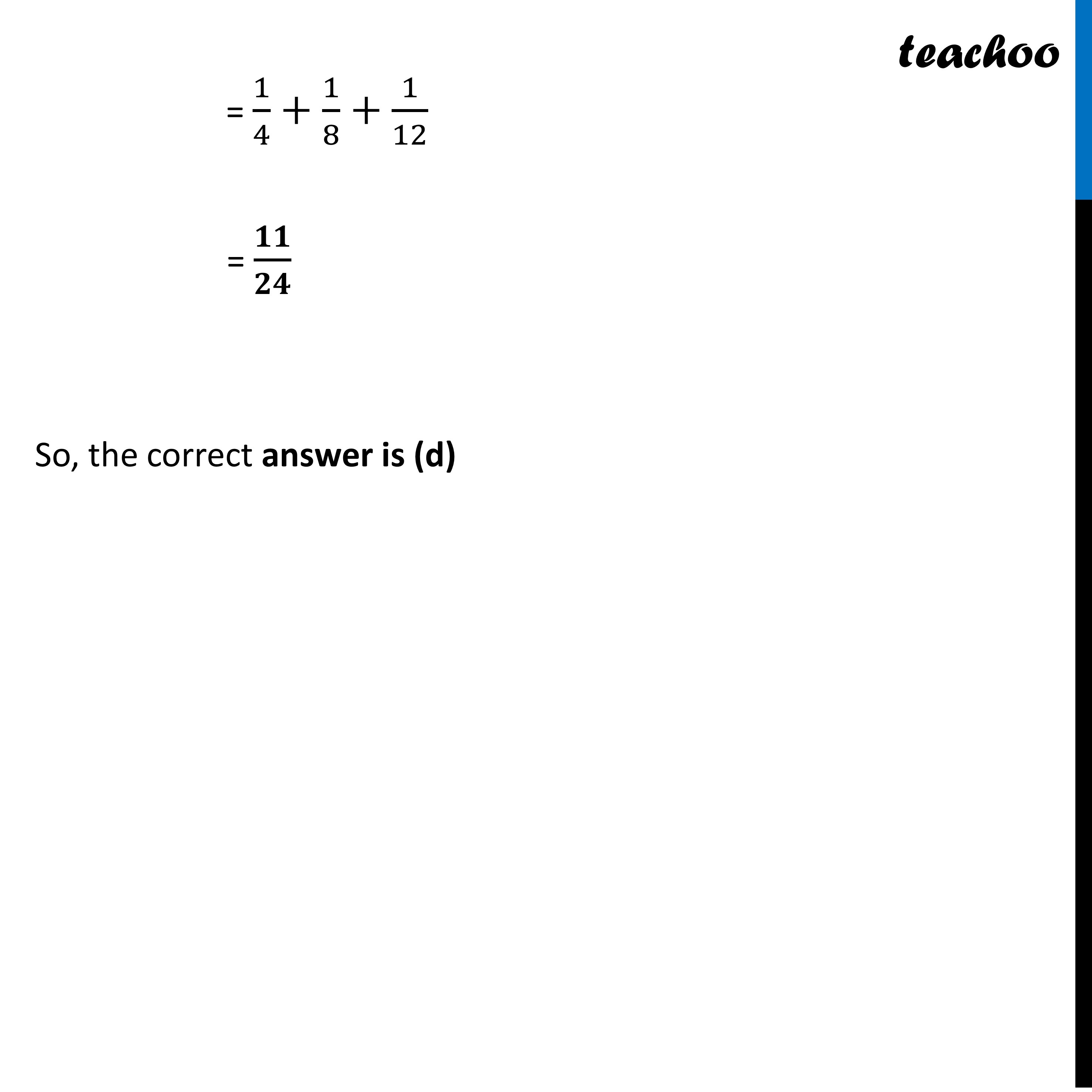## (D) 11/24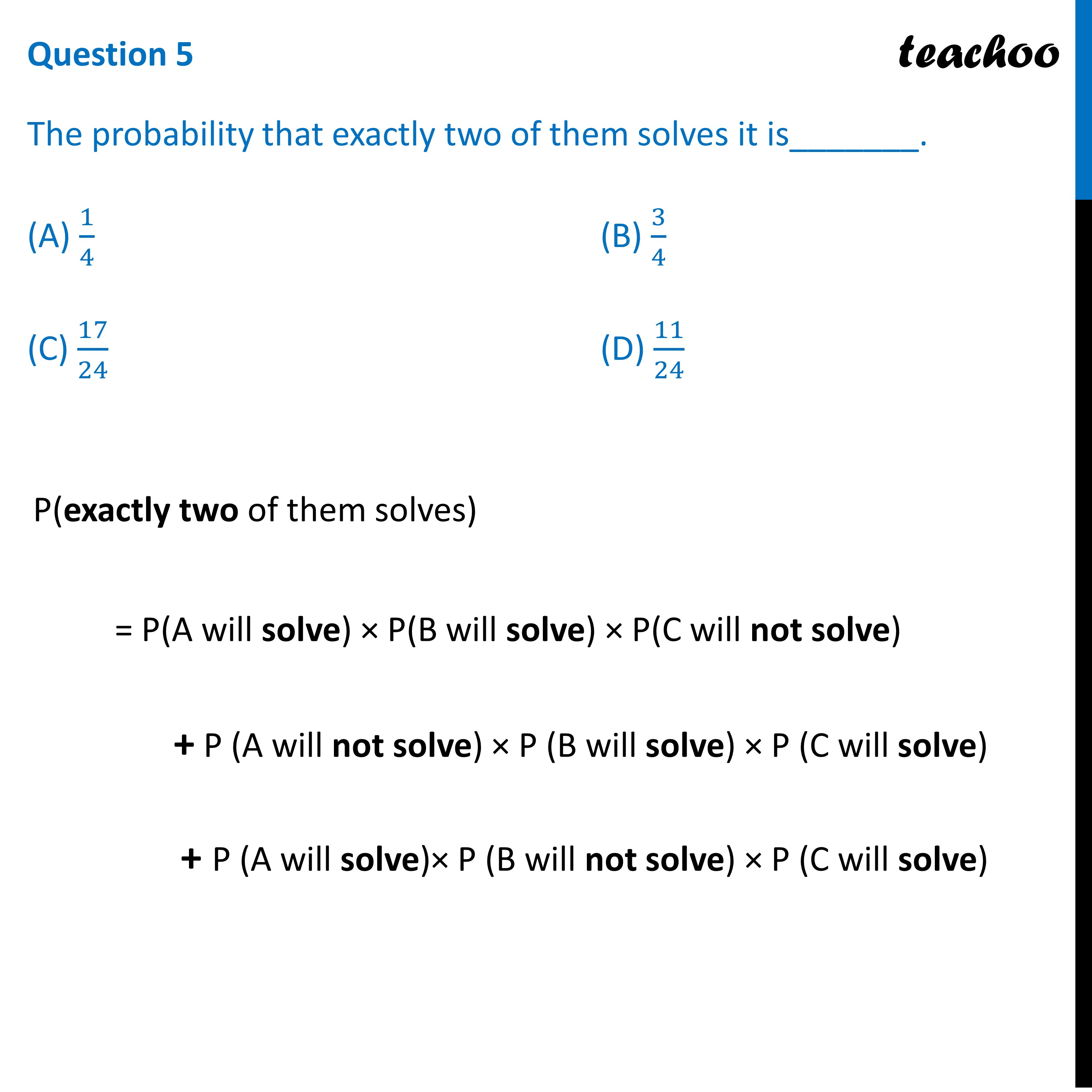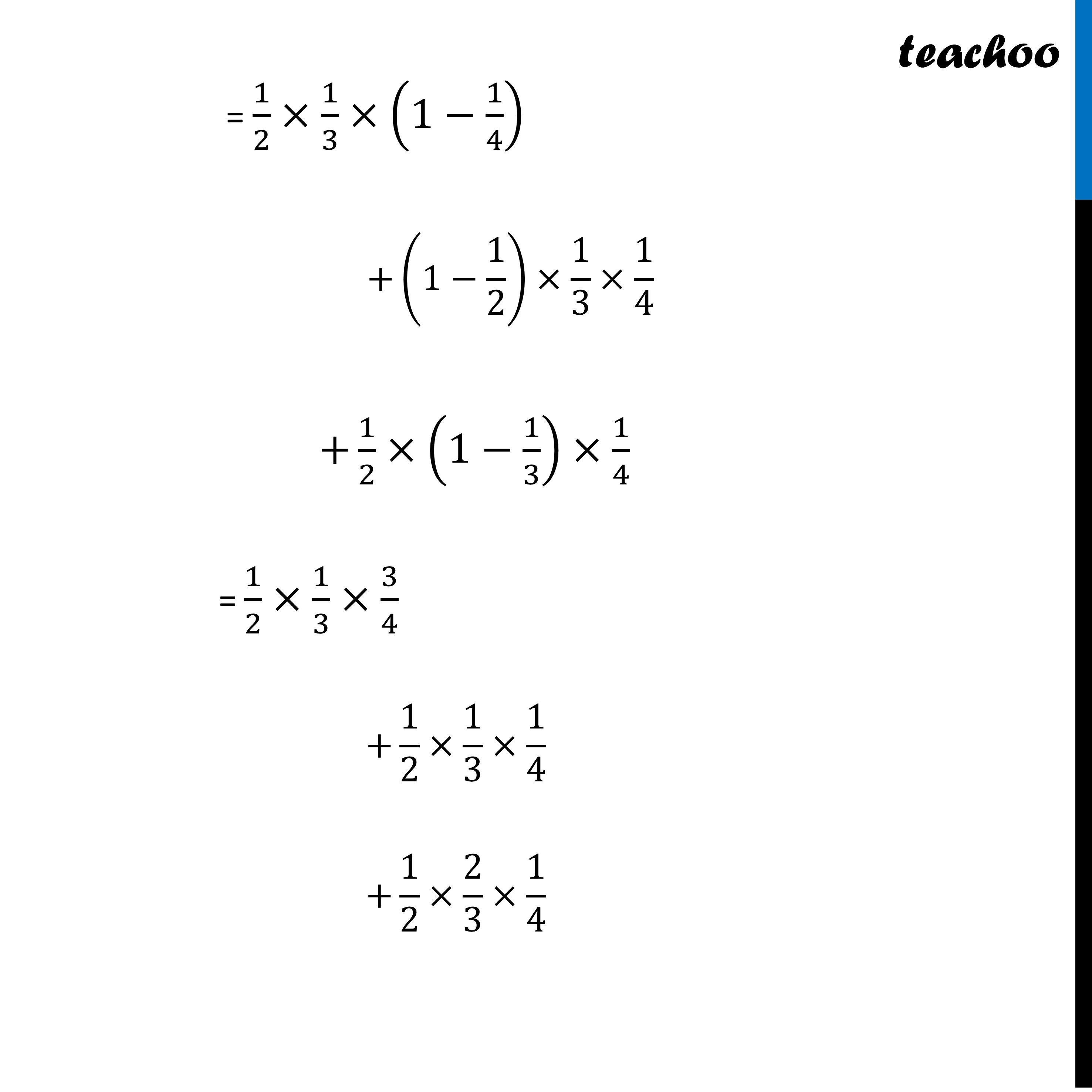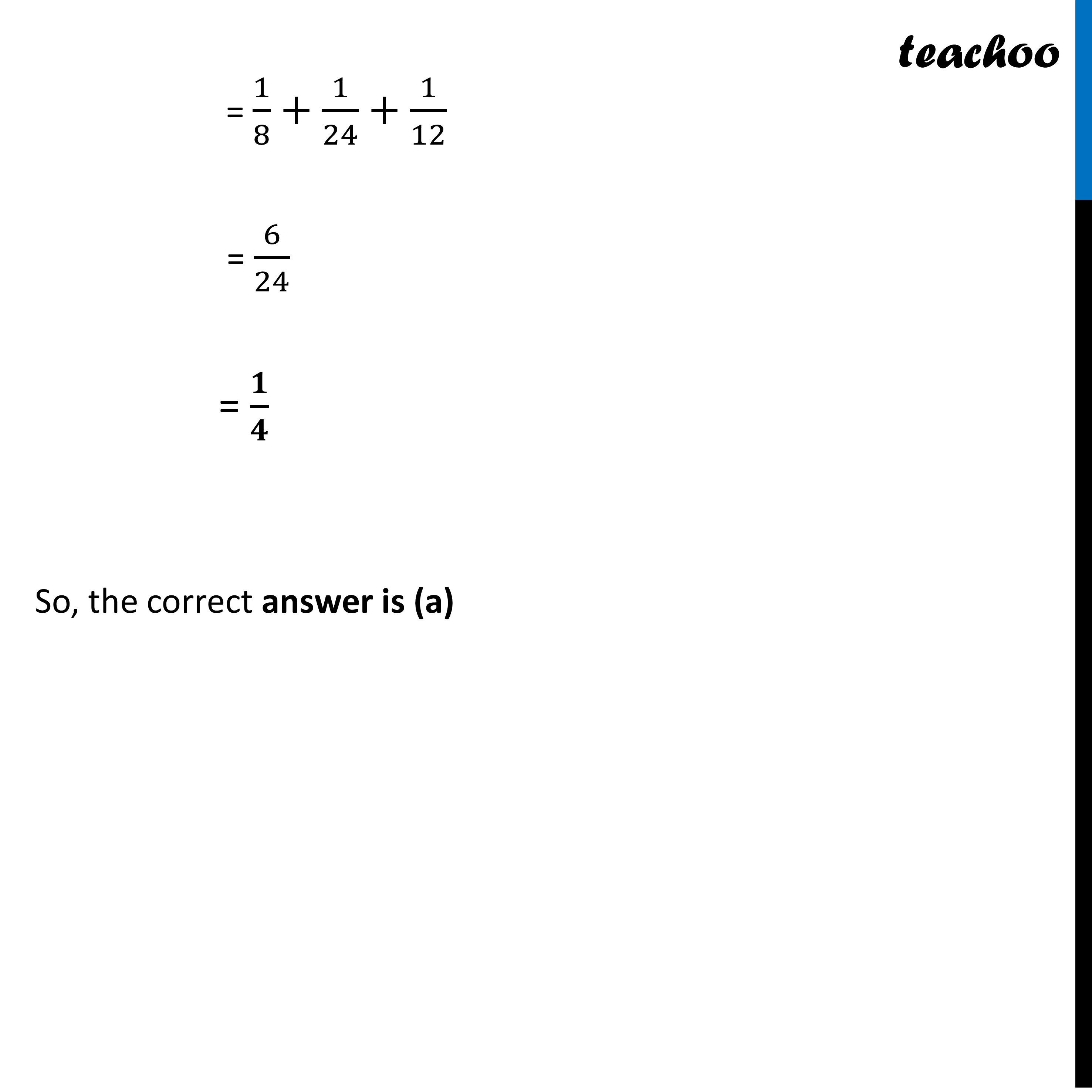Learn in your speed, with individual attention - Teachoo Maths 1-on-1 Class

### Transcript

Question Anand, Samanyu and Shah of SHORTCUTS classes were given a problem in Mathematics whose respective probabilities of solving it are 1/2, 1/3 and 1/4. They were asked to solve it independently. Based on the above data, answer any four of the following questions. Let, A : The event that Anand solves B : The event that Samanyu solves C : The event that Shah solves Given P(A) = 𝟏/𝟐 P(B) = 𝟏/𝟑 P(C) = 𝟏/𝟒 Question 1 The probability that Anand alone solves it is _______. (A) 1/4 (B) 3/4 (C) 11/24 (D) 17/24 P( A solves alone) = P(A solves) × P(B does not solve) × P(C does not solve) = 1/2×(1−1/3)×(1−1/4) = 1/2×2/3×3/4 = 𝟏/𝟒 So, the correct answer is (a) Question 2 The probability that problem is not solved is_______. (A) 1/4 (B) 3/4 (C) 0 (D) 11/24 P(problem is not solved) = P(A does not solve) × P(B does not solve) × P(C does not solve) = (1−1/2)×(1−1/3)×(1−1/4) = 1/2×2/3×3/4 = 𝟏/𝟒 So, the correct answer is (a) Question 3 The probability that the problem is solved is _______. (A) 1/4 (B) 3/4 (C) 17/24 (D) 11/24 P(problem is solved) = 1− P(problem is not solved) = 1 − 1/4 = 𝟑/𝟒 So, the correct answer is (b) Question 4 The probability that exactly one of them solves it is_______. (A) 1/4 (B) 3/4 (C) 17/24 (D) 11/24 P(exactly one of them solves) = P(A will solve) × P(B will not solve) × P(C will not solve) + P (A will not solve) × P (B will solve) × P (C will not solve) + P (A will not solve)× P (B will not solve) × P (C will solve) = 1/2×(1−1/3)×(1−1/4) +(1−1/2)×1/3×(1−1/4) +(1−1/2)×(1−1/3)×1/4 = 1/2×2/3×3/4 +1/2×1/3×3/4 +1/2×2/3×1/4 = 1/4+1/8+1/12 = 𝟏𝟏/𝟐𝟒 So, the correct answer is (d) Question 5 The probability that exactly two of them solves it is_______. (A) 1/4 (B) 3/4 (C) 17/24 (D) 11/24 P(exactly two of them solves) = P(A will solve) × P(B will solve) × P(C will not solve) + P (A will not solve) × P (B will solve) × P (C will solve) + P (A will solve)× P (B will not solve) × P (C will solve) = 1/2×1/3×(1−1/4) +(1−1/2)×1/3×1/4 +1/2×(1−1/3)×1/4 = 1/2×1/3×3/4 +1/2×1/3×1/4 +1/2×2/3×1/4 = 1/8+1/24+1/12 = 6/24 = 𝟏/𝟒 So, the correct answer is (a)